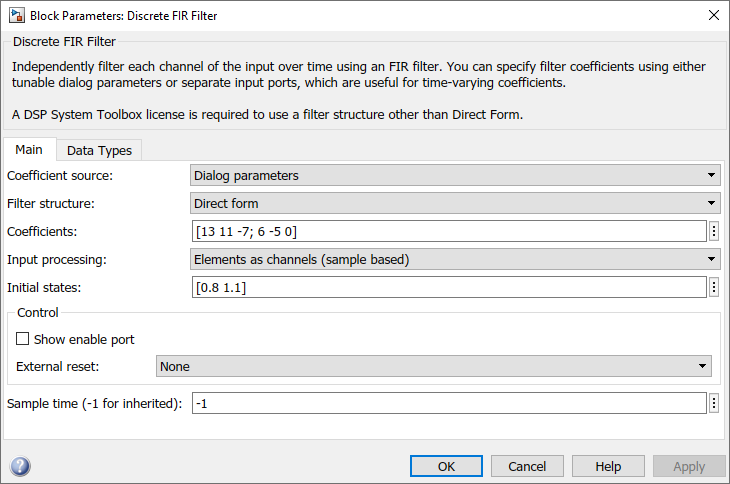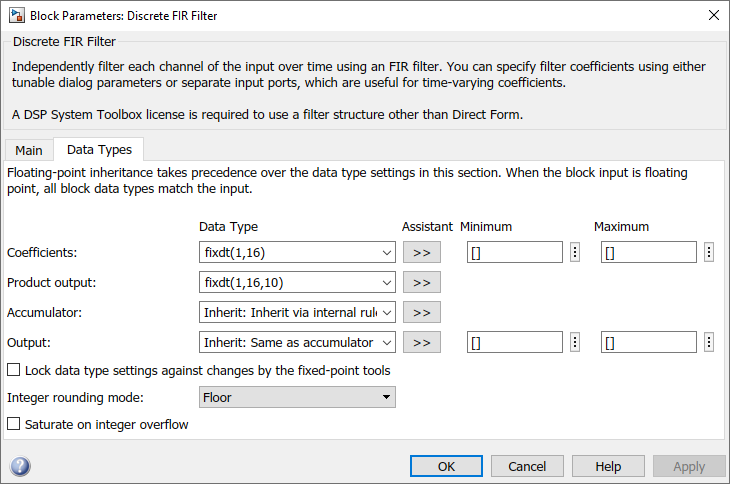## Conversions and Arithmetic Operations

This example uses the Discrete FIR Filter block to illustrate when parameters are converted from a double to a fixed-point number, when the input data type is converted to the output data type, and when the rules for addition, subtraction, and multiplication are applied.

Note

If a block can perform all four arithmetic operations, then the rules for multiplication and division are applied first. The Discrete FIR Filter block is an example of this.

Suppose you configure the Discrete FIR Filter block for two outputs, where the first output is given by

`${y}_{1}\left(k\right)=13\cdot u\left(k\right)+11\cdot u\left(k-1\right)-7\cdot u\left(k-2\right),$`

and the second output is given by

`${y}_{2}\left(k\right)=6\cdot u\left(k\right)-5\cdot u\left(k-1\right).$`

Additionally, the initial values of u(k–1) and u(k–2) are given by 0.8 and 1.1, respectively, and all inputs, parameters, and outputs have binary-point-only scaling.

To configure the Discrete FIR Filter block for this situation, on the Main pane of its dialog box, you must specify the Coefficients parameter as `[13 11 -7; 6 -5 0]` and the Initial states parameter as `[0.8 1.1]`, as shown here.Similarly, configure the options on the Data Types pane of the block dialog box to appear as follows:The Discrete FIR Filter block performs parameter conversions and block operations in the following order:

1. The Coefficients parameter is converted offline from doubles to the Coefficients data type using round-to-nearest and saturation.

The Initial states parameter is converted offline from doubles to the input data type using round-to-nearest and saturation.

2. The coefficients and inputs are multiplied together for the initial time step for both outputs. For y1(0), the operations 13·u(0), 11·0.8, and –7·1.1 are performed, while for y2(0), the operations u(0) and –5·0.8 are performed.

The results of these operations are stored as Product output.

3. The sum is carried out in Accumulator. The final summation result is then converted to Output.

4. Steps 2 and 3 repeat for subsequent time steps.